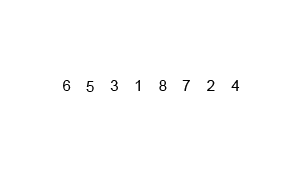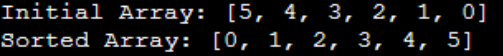×

Search anything:

# Implementing Merge Sort in Java

#### Java Sorting Algorithms AlgorithmsGet this book -> Problems on Array: For Interviews and Competitive Programming

# Concept

Merge Sort is a popular sorting algorithm that follows the divide-and-conquer paradigm. It efficiently sorts an array or a list by recursively dividing it into smaller subarrays, sorting them independently, and then merging them back together. The process continues until the entire array is sorted.

Merge sort has a time complexity of `O(n log n)`, making it an efficient sorting algorithm for large datasets. However, it requires additional space for temporary storage during the Dividing and Merging process, which is why it is considered a space-intensive algorithm.

In this article at OpenGenus, we have explored the design and implementation of Merge Sort in Java Programming Language.

# Example

Consider the following list of integers:

(2 4 1 6) (we want to sort it in ascending order)

Split the list into N sublists (step 1)
New list: (2) (4) (1) (6)

Step 2 and 3: Merge list in pairs of two.
Merge (2) (4) : (2 4)
Merge (1) (6) : (1 6)
New list: (2 4) (1 6)

Step 2: Merge the two lists (2 4) (1 6)
Compare 2 and 1: As 1 is smaller, it is inserted in the new list: (1)
Compare 2 and 6: As 2 is smaller, it is inserted in the new list: (1 2)
Compare 4 and 6: As 4 is smaller, it is inserted in the new list: (1 2 4)
Only one element 6 is left, so insert it in the new list: (1 2 4 6)

Entire list is sorted

## Visual RunThe merge step is the critical operation in merge sort. It involves comparing elements from the two sorted sublists and merging them into a sorted list.

Overall, merge sort is a stable, efficient, and reliable sorting algorithm used in various applications where a stable sort with good performance is needed.

# Algorithm

Follow these steps to sort any data using Merge Sort:

Step 1: Divide the unsorted list into n sublists; each sublist has one element (which is sorted as it has one element)

Step 2: Merge two lists at a time. While merging compare elements for the two sublists and prepare a new sorted list. This takes linear time.

Step 3: Do step 2 for all sub-list pairs.

Step 4: Repeat step 2 to 3 for the new list of sub-lists until we have only one list

# Implemented Code

1. Divide: In this function we break down the array into 2 equal halves until there is only a single element is left.
• We create mergeSort() function in which we pass an `ArrayList<Integer>` of integers.
``````import java.util.ArrayList;

class Main {
public static void mergeSort(ArrayList<Integer> nums){
``````
• This is a recursive function so a base case triggers if the length of the array we passed has a length of less than or equal to zero (meaning an array with single element or an empty array is already sorted.)
``````     //Setting the base case that if our array length is less than or equal to one.
//There is no need to merge.
if(nums.size()<=1)
return;
``````
• Next, we initialize mid to divide the array into 2 parts namely part1 and part2.
``````        //Now we divide our array into two parts.
//Handles both odd sized and even sized arrays.
int mid = nums.size()/2;
ArrayList<Integer> part1 = new ArrayList<Integer>(mid);
ArrayList<Integer> part2 = new ArrayList<Integer>(nums.size() - mid);
``````
• We only copy the elements from nums array to part1 which are less than mid. After that we copy the elements starting from mid to the nums length to part2.
``````     //Now we copy the elements from nums array to part1.
int x=0;
for(;x<mid;x++){
}

//Now we copy the left over elements from nums to part2.
for(; x<nums.size();x++){ //int x = part1.length
}
``````
• Now our nums array is successfully divided into two parts, part1 and part2.
• We keep calling merge sort on part1 and part2 until both are further divided and leaves a single element.
• Once there is no further division of array possible now we call to join function to join both the part1 and part2 arrays by comparing the elements present inside them and placing the least value element first.
• Final array of sorted nums is returned to the main function once the call Stack is empty.
• Please see the code below for a better understanding.
``````
//we call mergeSort on part1 and part2.
mergeSort(part1);
mergeSort(part2);

//we call our join function to join the two sorted arrays.
join(part1, part2, nums);
}
``````
1. Joining : In this function we compare the two sorted arrays and puts them into a single sorted array.
• In the join function we pass arrays part1, part2 and nums.
``````    public static void join(ArrayList<Integer> part1, ArrayList<Integer> part2, ArrayList<Integer> nums){
``````
• In this function we compare part1 and part2 arrays and puts the least element in the nums array. For this we initilaize i, x and y.
``````        int i = 0; //Index initialized for nums.
int x = 0; //Index initialized for part1.
int y = 0; //Index initialized for part2.
``````
• In case part1 and part2 both have the same element we first put part1's element in nums first and then part2's element. You can change this as per your choice.
``````        //We merge the two arrays part1 and part2 using while loop.
//While exits even if one of the arrays(part1 or part2) are fully copied into nums.
while(x<part1.size() && y<part2.size()){
//We compare both the array (part1 & part2) and the least element is placed first in the nums array.
if(part1.get(x)<=part2.get(y)){
nums.set(i, part1.get(x)); //Storing the element.
i++;//We increased the nums index after storing the element to accomadate the further element.
x++; //We incread the part1 index so that the next element can be compared.
}
//If the above condition fails, that means part2 has a lower element as compared to part1.
else{
nums.set(i, part2.get(y));
i++;
y++;
}
}
``````
• In case either part1 or part2 array are longer than the other one and after putting elements in nums there is still some element left in either of them, we then entirely copy that array's elements in the nums array.
• After comparing and putting elements into nums array the control is again returned to the mergeSort function.
• Please see the code below for better understanding.
``````        //In case part1 array's  size was greater than part2, we copy left over elements.
while(x<part1.size()){
nums.set(i, part1.get(x));
i++;
x++;
}

//In case part2 array's  size was greater than part1, we copy left over elements.
while(y<part2.size()){
nums.set(i, part2.get(y));
i++;
y++;
}
}
``````
1. main function: It is used to pass array to Merge Sort and prints the sorted array.
• We are using main function to call mergeSort on our nums array, which contains elements [5, 4, 3, 2, 1, 0]
• Using mergeSort function our array is transformed from [5, 4, 3, 2, 1, 0] ---> [0, 1, 2, 3, 4, 5]
• Please refer to the code below for better understanding.
``````   public static void main(String args[]){

ArrayList<Integer> nums = new ArrayList<Integer>();
System.out.println("Initial Array: "+nums);
mergeSort(nums);
System.out.println("Sorted Array: "+nums);

}
}
``````

# OUTPUT:# Complete Java Code

``````import java.util.ArrayList;

class Main {
public static void mergeSort(ArrayList<Integer> nums){
//Setting the base case that if our array length is less than or equal to one.
//There is no need to merge.
if(nums.size()<=1)
return;

//Now we divide our array into two parts.
//Handles both odd sized and even sized arrays.
int mid = nums.size()/2;
ArrayList<Integer> part1 = new ArrayList<Integer>(mid);
ArrayList<Integer> part2 = new ArrayList<Integer>(nums.size() - mid);

//Now we copy the elements from nums array to part1.
int x=0;
for(;x<mid;x++){
}

//Now we copy the left over elements from nums to part2.
for(; x<nums.size();x++){ //int x = part1.length
}

//we call mergeSort on part1 and part2.
mergeSort(part1);
mergeSort(part2);

//we call our join function to join the two sorted arrays.
join(part1, part2, nums);
}

public static void join(ArrayList<Integer> part1, ArrayList<Integer> part2, ArrayList<Integer> nums){
int i = 0; //Index initialized for nums.
int x = 0; //Index initialized for part1.
int y = 0; //Index initialized for part2.

//We merge the two arrays part1 and part2 using while loop.
//While exits even if one of the arrays(part1 or part2) are fully copied into nums.
while(x<part1.size() && y<part2.size()){
//We compare both the array (part1 & part2) and the least element is placed first in the nums array.
if(part1.get(x)<=part2.get(y)){
nums.set(i, part1.get(x)); //Storing the element.
i++;//We increased the nums index after storing the element to accomadate the further element.
x++; //We incread the part1 index so that the next element can be compared.
}
//If the above condition fails, that means part2 has a lower element as compared to part1.
else{
nums.set(i, part2.get(y));
i++;
y++;
}
}

//In case part1 array's  size was greater than part2, we copy left over elements.
while(x<part1.size()){
nums.set(i, part1.get(x));
i++;
x++;
}

//In case part2 array's  size was greater than part1, we copy left over elements.
while(y<part2.size()){
nums.set(i, part2.get(y));
i++;
y++;
}
}
public static void main(String args[]){

ArrayList<Integer> nums = new ArrayList<Integer>();
System.out.println("Initial Array: "+nums);
mergeSort(nums);
System.out.println("Sorted Array: "+nums);

}
}
``````

With this article at OpenGenus, you must have the complete idea of implementing Merge Sort in Java Programming Language.